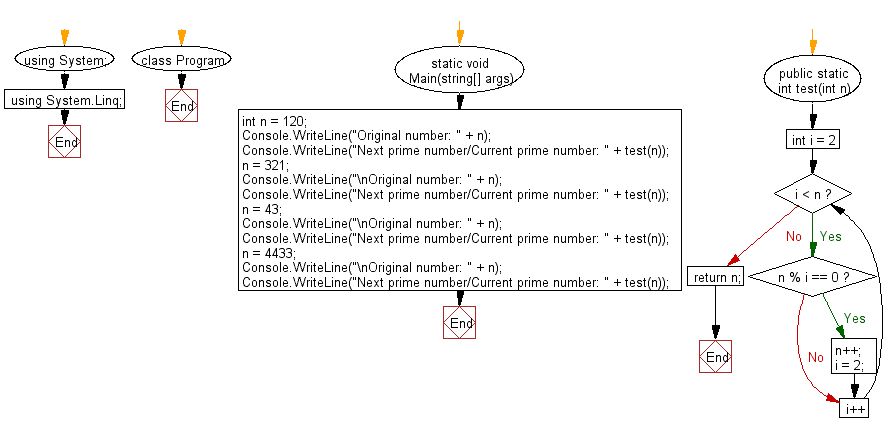﻿ C# - Next prime number of a given integer

# C#: Next prime number of a given integer

## C# Sharp Basic: Exercise-92 with Solution

Write a C# Sharp program to find the next prime number of a given number. If the given number is a prime number, return the number.

From Wikipedia,
A prime number (or a prime) is a natural number greater than 1 that is not a product of two smaller natural numbers. A natural number greater than 1 that is not prime is called a composite number. For example, 5 is prime because the only ways of writing it as a product, 1 × 5 or 5 × 1, involve 5 itself. However, 4 is composite because it is a product (2 × 2) in which both numbers are smaller than 4. Primes are central in number theory because of the fundamental theorem of arithmetic: every natural number greater than 1 is either a prime itself or can be factorized as a product of primes that is unique up to their order.

Sample Solution:

C# Sharp Code:

``````using System;

namespace exercises
{
class Program
{
static void Main(string[] args)
{
// Initializing integer 'n' with various values
int n = 120;
Console.WriteLine("Original number: " + n);

// Displaying the next prime number or the current prime number of 'n'
Console.WriteLine("Next prime number/Current prime number: " + test(n));

// Changing the value of 'n' and repeating the process
n = 321;
Console.WriteLine("\nOriginal number: " + n);
Console.WriteLine("Next prime number/Current prime number: " + test(n));

// Changing the value of 'n' and repeating the process
n = 43;
Console.WriteLine("\nOriginal number: " + n);
Console.WriteLine("Next prime number/Current prime number: " + test(n));

// Changing the value of 'n' and repeating the process
n = 4433;
Console.WriteLine("\nOriginal number: " + n);
Console.WriteLine("Next prime number/Current prime number: " + test(n));
}

// Method to find the next prime number or the current prime number of a given number 'n'
public static int test(int n)
{
for (int i = 2; i < n; i++)
{
// Checking if 'n' is divisible by 'i', if true, increment 'n' and reset 'i' to 2
if (n % i == 0) {
n++;
i = 2;
}
}
return n; // Returning the next or current prime number
}
}
}
``````

Sample Output:

```Original number: 120
Next prime number/Current prime number: 127

Original number: 321
Next prime number/Current prime number: 331

Original number: 43
Next prime number/Current prime number: 43

Original number: 4433
Next prime number/Current prime number: 4441
```

Flowchart:C# Sharp Code Editor:

Previous C# Sharp Exercise: Write a C# Sharp program to remove all the values except integer values from a given array of mixed values.
Next C# Sharp Exercise: Square root of a given number.

What is the difficulty level of this exercise?

﻿